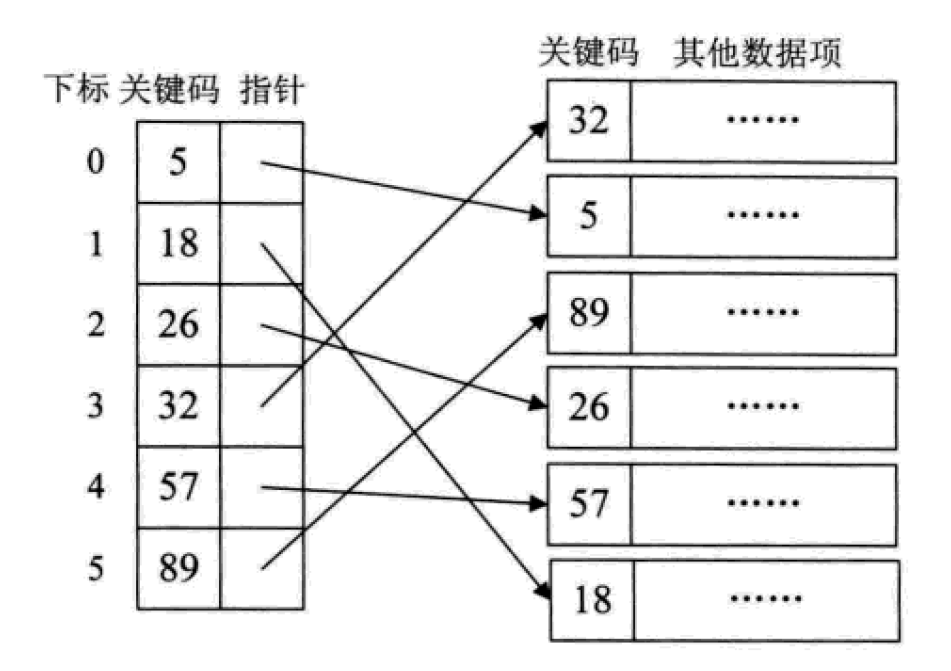Python中常用的查找数据结构及算法汇总# 最基础的遍历无序列表的查找算法# 时间复杂度O(n)
def sequential_search(lis, key):
length = len(lis)
for i in range(length):
if lis[i] == key:
return i
else:
return False
if __name__ == '__main__':
LIST = [1, 5, 8, 123, 22, 54, 7, 99, 300, 222]
result = sequential_search(LIST, 123)
print(result)

1. 二分查找(Binary Search)

# 针对有序查找表的二分查找算法# 时间复杂度O(log(n))
def binary_search(lis, key):
low = 0
high = len(lis) - 1
time = 0
while low < high:
time += 1
mid = int((low + high) / 2)        if key < lis[mid]:
high = mid - 1
elif key > lis[mid]:
low = mid + 1
else:            # 打印折半的次数
print("times: %s" % time)            return mid
print("times: %s" % time)    return Falseif __name__ == '__main__':
LIST = [1, 5, 7, 8, 22, 54, 99, 123, 200, 222, 444]
result = binary_search(LIST, 99)
print(result)

2. 插值查找

value = (key – list[low])/(list[high] – list[low])

# 插值查找算法# 时间复杂度O(log(n))
def binary_search(lis, key):
low = 0
high = len(lis) - 1
time = 0
while low < high:
time += 1
# 计算mid值是插值算法的核心代码
mid = low + int((high - low) * (key - lis[low])/(lis[high] - lis[low]))
print("mid=%s, low=%s, high=%s" % (mid, low, high))        if key < lis[mid]:
high = mid - 1
elif key > lis[mid]:
low = mid + 1
else:            # 打印查找的次数
print("times: %s" % time)            return mid
print("times: %s" % time)    return Falseif __name__ == '__main__':
LIST = [1, 5, 7, 8, 22, 54, 99, 123, 200, 222, 444]
result = binary_search(LIST, 444)
print(result)

3. 斐波那契查找

F = [1, 1, 2, 3, 5, 8, 13, 21, 34, 55, 89, 144,…]

# 斐波那契查找算法# 时间复杂度O(log(n))def fibonacci_search(lis, key):
# 需要一个现成的斐波那契列表。其最大元素的值必须超过查找表中元素个数的数值。
F = [1, 1, 2, 3, 5, 8, 13, 21, 34, 55, 89, 144,         233, 377, 610, 987, 1597, 2584, 4181, 6765,         10946, 17711, 28657, 46368]
low = 0
high = len(lis) - 1

# 为了使得查找表满足斐波那契特性，在表的最后添加几个同样的值
# 这个值是原查找表的最后那个元素的值
# 添加的个数由F[k]-1-high决定
k = 0
while high > F[k]-1:
k += 1
print(k)
i = high    while F[k]-1 > i:
lis.append(lis[high])
i += 1
print(lis)
# 算法主逻辑。time用于展示循环的次数。
time = 0
while low <= high:
time += 1
# 为了防止F列表下标溢出，设置if和else
if k < 2:
mid = low        else:
mid = low + F[k-1]-1

print("low=%s, mid=%s, high=%s" % (low, mid, high))        if key < lis[mid]:
high = mid - 1
k -= 1
elif key > lis[mid]:
low = mid + 1
k -= 2
else:            if mid <= high:                # 打印查找的次数
print("times: %s" % time)                return mid            else:
print("times: %s" % time)                return high
print("times: %s" % time)    return Falseif __name__ == '__main__':
LIST = [1, 5, 7, 8, 22, 54, 99, 123, 200, 222, 444]
result = fibonacci_search(LIST, 444)
print(result)Python开发开发项目